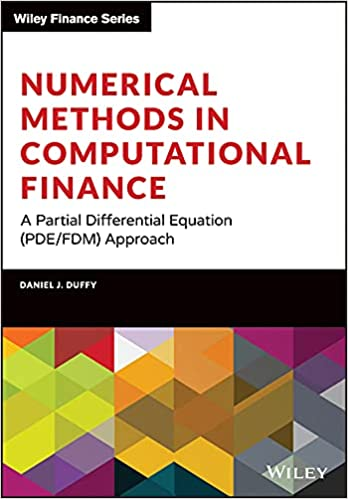# Numerical Methods in Computational Finance: A Part ial Differential Equation (PDE/FDM) Approach (Wiley Finance)

This book is a detailed and step-by-step introduction to the mathematical foundations of ordinary and partial differential equations, their approximation by the finite difference method and applications to computational finance. The book is structured so that it can be read by beginners, novices and expert users.

Part A Mathematical Foundation for One-Factor Problems

Chapters 1 to 7 introduce the mathematical and numerical analysis concepts that are needed to understand the finite difference method and its application to computational finance.

Part B Mathematical Foundation for Two-Factor Problems

Chapters 8 to 13 discuss a number of rigorous mathematical techniques relating to elliptic and parabolic partial differential equations in two space variables. In particular, we develop strategies to preprocess and modify a PDE before we approximate it by the finite difference method, thus avoiding ad-hoc and heuristic tricks.

Part C The Foundations of the Finite Difference Method (FDM)

Chapters 14 to 17 introduce the mathematical background to the finite difference method for initial boundary value problems for parabolic PDEs. It encapsulates all the background information to construct stable and accurate finite difference schemes.

Part D Advanced Finite Difference Schemes for Two-Factor Problems

Chapters 18 to 22 introduce a number of modern finite difference methods to approximate the solution of two factor partial differential equations. This is the only book we know of that discusses these methods in any detail.

Part E Test Cases in Computational Finance

Chapters 23 to 26 are concerned with applications based on previous chapters. We discuss finite difference schemes for a wide range of one-factor and two-factor problems.

This book is suitable as an entry-level introduction as well as a detailed treatment of modern methods as used by industry quants and MSc/MFE students in finance. The topics have applications to numerical analysis, science and engineering.

More on computational finance and the author’s online courses, see www.datasim.nl.

Price: £49.51

Shipping: £0.00